# Energy Decomposition Analysis (EDA)¶

## Energy Decomposition Analysis of Ammonia Borane¶

This tutorial explains how to perform an energy decomposition analysis (EDA)  of Ammonia borane, in which ammonia and borane have a donor-acceptor interaction with each other. Hereby, the user can examine which interactions result in a stable complex.

The bond energy of H3N-BH3 is defined as:

$\Delta E = E_{NH_3-BH_3} - E_{NH_3} -E_{BH_3}$

in which ENH3 and EBH3 are the energies of the optimized reactants, and EH3N-BH3 is the energy of the optimized complex. The bond energy consists of the preparation energy, also known as the strain energy, and the interaction energy:

$\Delta E = \Delta E_{prep} + \Delta E_{int}$

The preparation energy is the amount of energy that is required to deform the NH3 and BH3 from their equilibrium structure to the geometry they have in the complex. The interaction energy is the change in energy when the prepared fragments (NH3 and BH3 in the complex geometry) are combined to form the complex. A quantitative energy decomposition analysis (EDA) divides the interaction energy in the electrostatic interaction, Pauli repulsion, and attractive orbital interactions:

$\Delta E_{int} = \Delta V_{elstat} + \Delta E_{Pauli} + \Delta E_{oi}$

The electrostatic interaction, which is usually attractive, is the energy between the unperturbed charge distributions of the prepared fragments. The Pauli repulsion is responsible for steric repulsion, it consists of the destablilizing interactions between occupied orbitals of the fragments. The orbital interaction accounts for charge transfer and polarization.

If you want to do an EDA with unrestricted fragments, see the following tutorial instead.

### Geometry Optimization¶

The geometry of the reactants and the product (complex) must be optimized. Note that another basis set and functional can be used as well. Symmetry will be used, which may help in the analysis.

Perform the following steps for NH3, BH3 and H3N-BH3:

1. In AMSinput build the structure (or search for it in the search box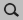)
2. Symmetrize the system by clicking on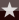3. Select TaskGeometry Optimization
4. Select XC functionalGGA:BP86
5. Select Basis setTZP
5. Select Numerical qualityGood
7. Run the calculation with FileRun (give an appropriate name to your calculations)
8. When the run has finished, click ‘Yes’ to import the optimized coordinates and save it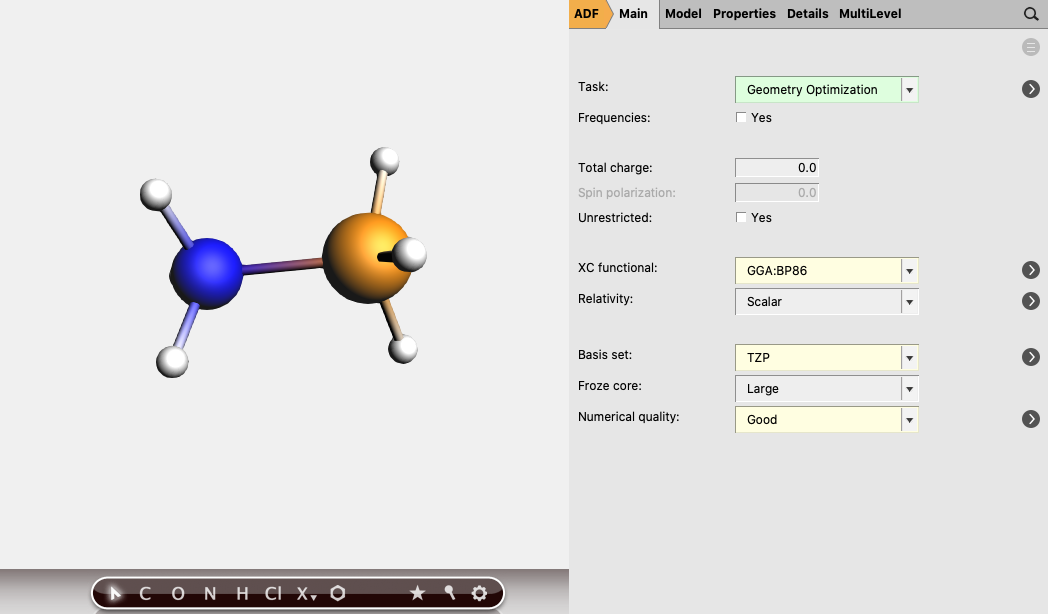The bond energy of H3N-BH3 can be calculated by subtracting the energies of the reactants, NH3 and BH3 from the energy of the complex. The energies can be found at the bottom of the logfile or in the output.

$\Delta E = E_{H_3N-BH_3} - E_{NH_3} -E_{BH_3}$

The bond energy of H3N-BH3 was calculated to be -31.80 kcal/mol with these settings. (-838.47 - (-444.53) - (-362.14) = -31.80)

### EDA¶

Single point calculations of the prepared fragments NH3 and BH3 are needed to perform an EDA. In the GUI, single points of the fragments will be computed automatically when you follow the next steps for a fragment analysis:

Perform a new calculation using the geometry of the optimized complex (H3N-BH3):
1. Select TaskSingle Point
2. Select XC functionalGGA:BP86
3. Select Basis setTZP
4. Select Numerical qualityGood
5. Go in the panel bar to ModelRegions
6. Select the atoms of NH3 and click the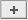button next to Regions
7. Select the atoms of BH3 and click also on thebutton8. Go in the panel bar to MultiLevelFragments
9. Check the ‘Use fragments’ check box
10. Run the calculation

When doing calculations on a cluster, single point calculations of NH3 and BH3 in their prepared geometry must be performed at first. The adf.rkf files of the single point computations are needed for the fragment analyses.

### Analysis¶

The energy decomposition of the interaction energy of H3N-BH3 can be found in the output file of the fragment analysis. You can find for instance that H3N-BH3 has an interaction energy of -44.63 kcal/mol, which consists of a Pauli repulsion of 108.10 kcal/mol, an electrostatic interaction of -77.53 kcal/mol, and an orbital interaction of -75.19 kcal/mol. This results in an interaction energy of -44.63 kcal/mol.

The orbital interaction is decomposed into contributions from different irreducible representations of the molecular point group. In this case the contributions from A1 ($$\sigma, \sigma^*$$-orbitals) are much more important than those from E1 ($$\pi, \pi^*$$-orbitals).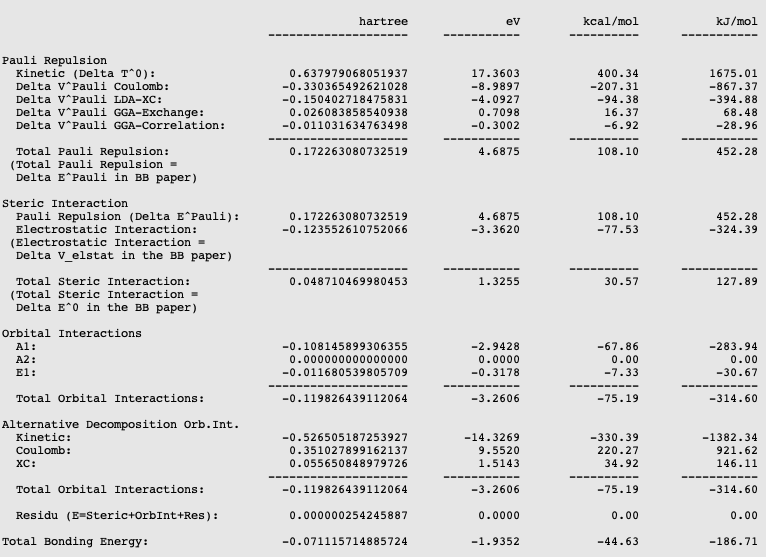The bond energy was calculated previously to be -31.80 kcal/mol and accordingly the preparation energy can be calculated to be 12.83 kcal/mol (=-31.80-(-44.63)). The structure of the fully relaxed NH3 is only slightly different from the structure of the NH3 fragment in H3N-BH3. However, the structure of the fully relaxed flat BH3 (symmetry D3h) is substantially different than the structure of the BH3 trigonal pyramidal fragment in H3N-BH3 (symmetry C3v):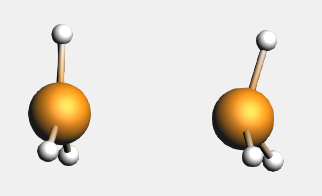The preparation energy for NH3 is only 0.11 kcal/mol, the difference between the bond energy of the NH3 fragment (-444.42 kcal/mol) and the fully relaxed NH3 molecule (-444.53 kcal/mol). Due to the relatively large structural changes the preparation energy for BH3 is much larger, namely 12.72 kcal/mol, the difference between the bond energy of the BH3 trigonal pyramidal fragment (-349.42 kcal/mol) and the fully relaxed planar BH3 molecule (-362.14 kcal/mol). Note that the energies of the fragments can be found at the bottom of the logfile or in the output of the fragments.

With AMSlevels the molecular orbital diagram can be visualized, in which one can see a donor-acceptor interaction and (repulsive) interactions between occupied orbitals.

1. SCM → Levels
2. In AMSlevels, click on View → Labels → Show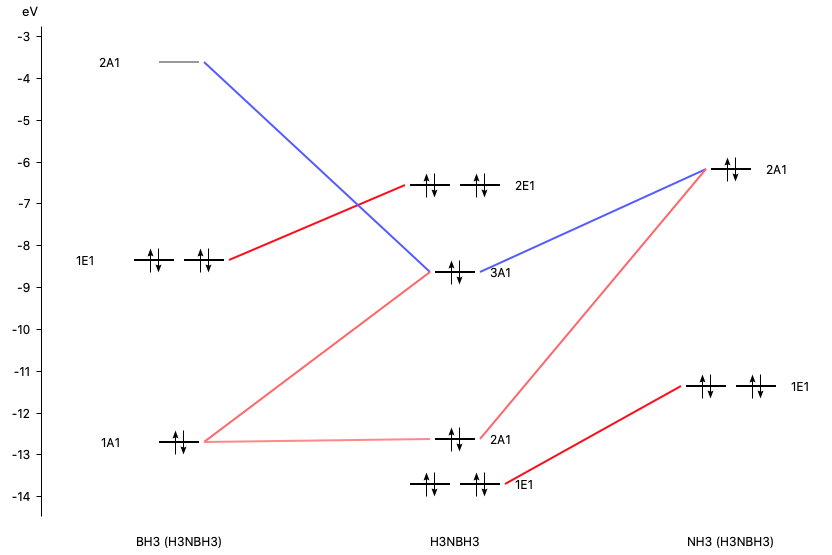By right clicking the BH3 2A1 fragment orbital (LUMO of BH3, acceptor orbital) one can select the corresponding SFO (fragment orbital), which can be visualized with AMSview. Similar one can select the NH3 2A1 fragment orbital (HOMO of NH3, donor orbital). After some manipulations, using 50% opacity, one can get the following two AMSview windows that show these fragment orbitals.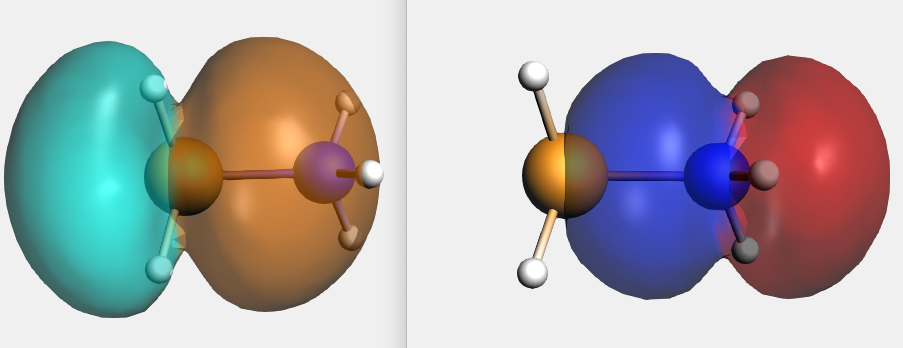## EDA with unrestricted fragments¶

This tutorial explains how to perform an energy decomposition analysis (EDA) of a molecule with unrestricted fragments, for example the CH3 groups of ethane. Hereby, the user can examine which interactions result in the stable molecule. To see an explanation of these different interactions or to do an EDA without unrestricted fragments, see the EDA of Ammonia Borane tutorial.

### Geometry Optimization¶

The geometry of the reactants and the product (complex) must be optimized. Note that another basis set and functional can be used as well. . Symmetry will be used, which may help in the analysis.

For ethane:

1. In AMSinput make the structure or search for the structure in the search boxin the panel bar
2. Symmetrize the system by clicking on3. Select TaskGeometry Optimization
4. Select XC functionalGGA:BP86
5. Select Basis setTZP
5. Select Numerical qualityGood
7. Run the calculation with FileRun (give an appropriate name to your calculations)
8. When the run has finished, click ‘Yes’ to import the optimized coordinates and save it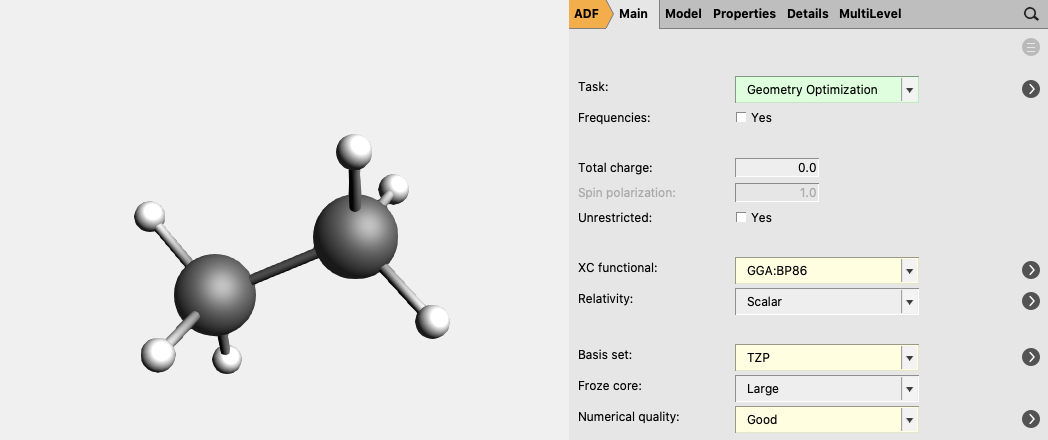For CH3 (methyl) an unrestricted calculation is needed:

1. In AMSinput make the structure or search for the structure in the search boxin the panel bar
2. Symmetrize the system by clicking on3. Select TaskGeometry Optimization
4. Check the Unrestricted box.
5. Enter 1.0 as Spin polarization
6. Select XC functionalGGA:BP86
7. Select Basis setTZP
8. Select Numerical qualityGood
9. Run the calculation with FileRun (give an appropriate name to your calculations)
10. When the run has finished, click ‘Yes’ to import the optimized coordinates and save it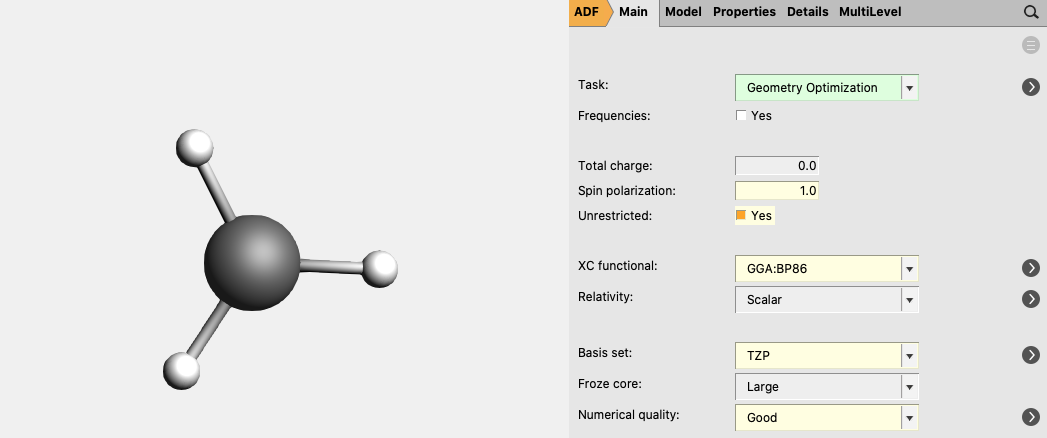The bond energy of C2H6 can be calculated by subtracting the energies of the reactants (two CH3) from the energy of the complex. The energies can be found at the bottom of the logfile or in the output.

$\Delta E = E_{C_2H_6} - 2 E_{CH_3}$

The bond energy of C2H6 was calculated to be -93.53 kcal/mol with these settings. (-920.23 - 2*(-413.35)) = -93.53)

### EDA¶

Single point calculations of the CH3 groups in the geometry they have in the product are needed to perform an EDA. Note that one sometimes need to change the electron configuration of the fragments to make them so called ‘prepared for bonding’ in order to minimize the Pauli repulsion in the electron pair bond. This is not needed in this simple example.

1. Perform a new calculation with the optimized molecule, ethane
2. Select the Task Single Point
3. Check the Unrestricted box.
4. Select XC functionalGGA:BP86
5. Select Basis setTZP
6. Select Numerical qualityGood
7. Go in the panel bar to ModelRegions
8. Select the atoms of one CH3 group and click thebutton next to Regions
9. Select the atoms of the other CH3 group and click also on thebutton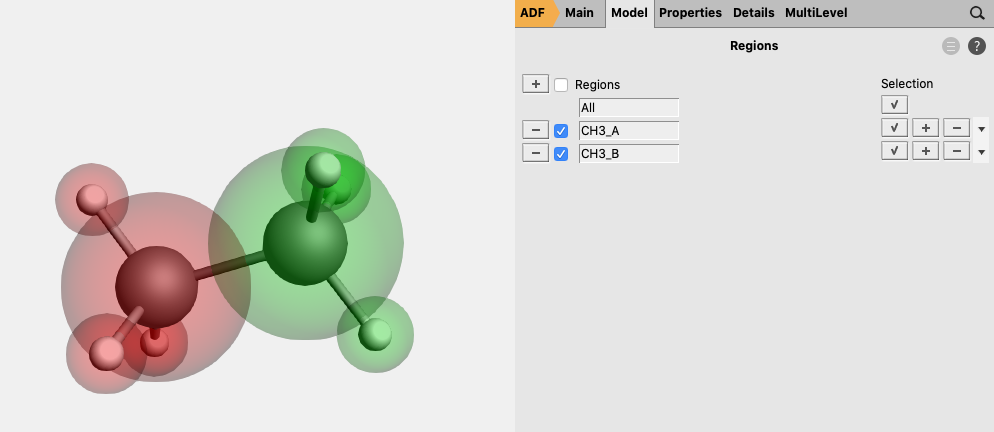10. Go in the panel bar to MultiLevelFragments
11. Check the ‘Use fragments’ check box (A warning will popup regarding NOSYM symmetry)
12. Enter as spin 1 for one of the fragments and -1 for the other fragment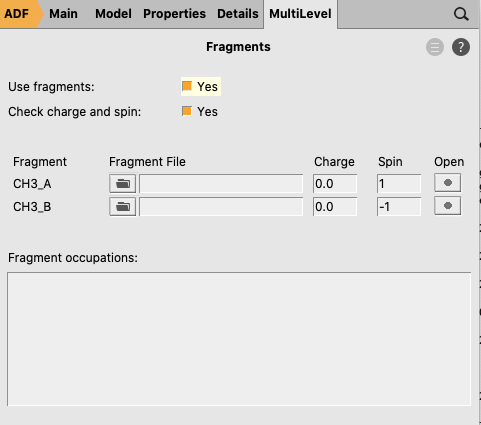The symmetry has been adjusted to NOSYM. However, we want to use symmetry. Besides setting the symmetry to AUTO, in this case we also need to symmetrize the coordinates again, since using fragments will lower the symmetry of ethane that ADF can use from D3d to C3v. Note that in this case the symmetrization will only reorient the geometry in order to fulfill the molecular orientation requirements in ADF such that ADF can use symmetry.

13. Go in the panel bar to DetailsSymmetry
14. Select SymmetryAUTO
15. Symmetrize the system by clicking on16. Save it and run the calculation

### Analysis¶

The different energies, where the interaction energy of the CH3 groups of ethane consists of, are noted in the output. It can be noticed that the interaction energy of -111.41 kcal/mol is build out of 180.02 kcal/mol Pauli repulsion, -125.43 kcal/mol electrostatic interaction, and -166.02 kcal/mol orbital interactions.

The orbital interaction is decomposed into contributions from different irreducible representations of the molecular point group. In this case the contributions from A1 ($$\sigma, \sigma^*$$-orbitals) are much more important than those from E1 ($$\pi, \pi^*$$-orbitals).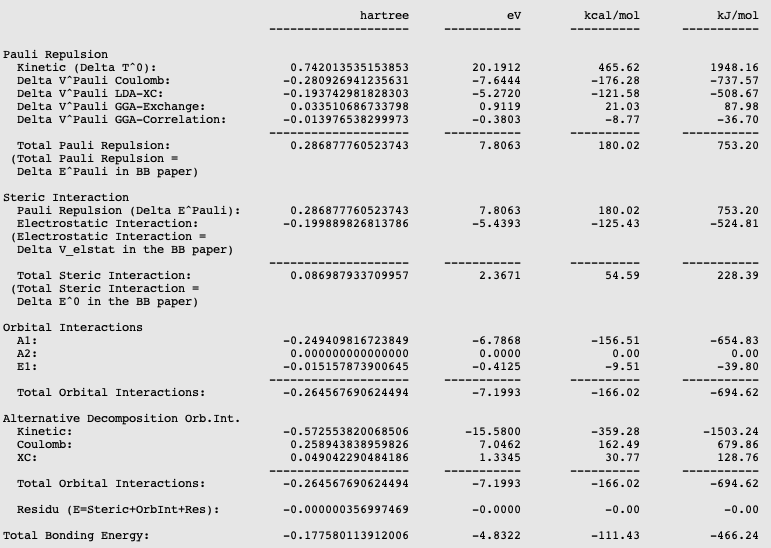The bond energy was calculated previously to be -93.53 kcal/mol and accordingly the preparation energy can be calculated to be 17.88 kcal/mol (=-93.53-(-111.41)). The preparation energy for one CH3 fragment is 8.95 kcal/mol, the difference between the bond energy of the CH3 trigonal pyramidal fragment (-404.40 kcal/mol) and the fully relaxed planar CH3 molecule (-413.35 kcal/mol). The preparation energy of the other CH3 fragment is the same. Note that the energies of the fragments can be found at the bottom of the logfile or in the output of the fragments.

With AMSlevels the molecular orbital diagram can be visualized, in which one can see an electron-pair bond and (repulsive) interactions between occupied levels.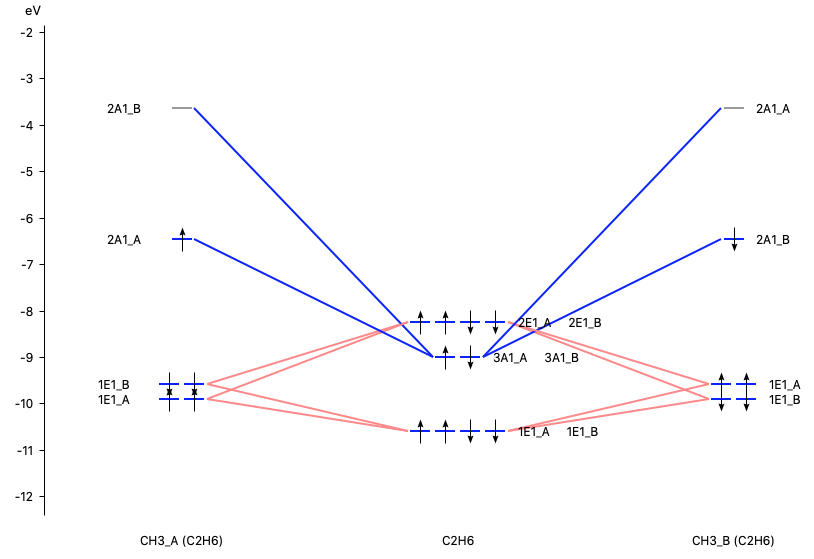F.M. Bickelhaupt, E.J. Baerends J.P., Kohn-Sham Density Functional Theory: Predicting and Understanding Chemistry, Reviews in Computational Chemistry 15, 1 (2000)